# How much water would be needed to completely dissolve 1.74 L of the gas at a...

How much water would be needed to completely dissolve 1.74 L of the gas at a pressure of 725 torr and a temperature of 18 ∘C?

A gas has a Henry's law constant of 0.192 M/atm .

Answer: Here we have to use the henry's law

C = KP , here , C is concentration , K is henry's constant and P is partial pressure

given mass / molar mass of water* volume = 0.192 * pressure in atm

[ here 1 torr = 0.0013atm hence , 725 torr = 0.95 atm ]

Now , we get : mass / 18 * 1.74 = 0.95 * 0.192

mass = 5.71 gram

Hence the required amount of water is 5.71 gram

##### Add Answer to: How much water would be needed to completely dissolve 1.74 L of the gas at a...
Similar Homework Help Questions
• ### If 108.1 mL of water is shaken with a gas at 2.94 atm, it will dissolve 0.00270 g of the gas....

If 108.1 mL of water is shaken with a gas at 2.94 atm, it will dissolve 0.00270 g of the gas. Estimate the Henry's law constant for the gas in water in units of g L-1atm-1.

• ### Determine the mass (in grams) of N, that will dissolve in 155 L of water that...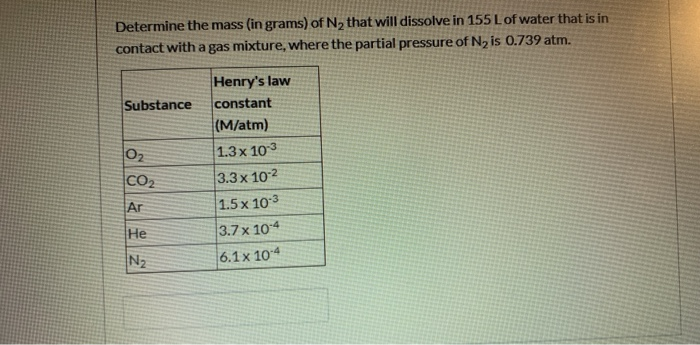Determine the mass (in grams) of N, that will dissolve in 155 L of water that is in contact with a gas mixture, where the partial pressure of N is 0.739 atm. Substance Henry's law constant (M/atm) 1.3 x 103 3.3 x 10-2 1.5 x 10-3 3.7 x 10-4 6.1 x 10-4 Ng

• ### Henry's Law states that the quantity of a gas that will dissolve in a liquid is...

Henry's Law states that the quantity of a gas that will dissolve in a liquid is proportional to the partial pressure of the gas and its solubility coefficient (its physical or chemical attraction for water), at a given temperature. Henry's Law: The volume of a gas (Vx) dissolved in a liter of water is Vx = (pX)*(SC), where pX is the partial pressure in atmospheres and SC is the solubility coefficient. a) At 1 atm and 37 degrees Celsius, the...

• ### Determine the mass (in grams) of N2 that will dissolve in 155 L of water that...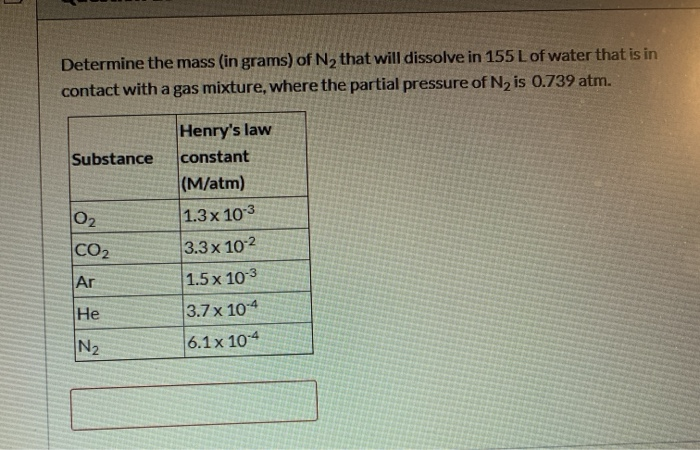Determine the mass (in grams) of N2 that will dissolve in 155 L of water that is in contact with a gas mixture, where the partial pressure of N, is 0.739 atm. Henry's law constant Substance (M/atm) 1.3 x 103 3.3 x 102 1.5 x 10-3 3.7 x 10-4 Ar He N2 6.1 x 10-4

• ### molality

How much water would be needed to completely dissolve 1.66 {rm L} of the gas at a pressure of 745 {rm torr} and a temperature of 22^circ {rm C}?

• ### 9. Henry's Law states that the quantity of a gas that will dissolve in a liquid...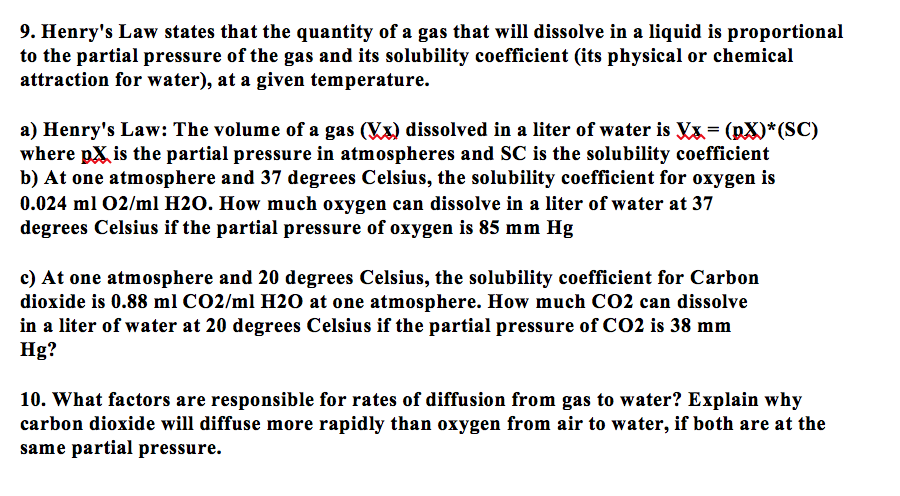9. Henry's Law states that the quantity of a gas that will dissolve in a liquid is proportional to the partial pressure of the gas and its solubility coefficient (its physical or chemical attraction for water), at a given temperature a) Henry's Law: The volume of a gas (Vx) dissolved in a liter of water is Vx= (p3)*(SC) where pX is the partial pressure in atmospheres and SC is the solubility coefficient b) At one atmosphere and 37 degrees Celsius,...

• ### Henry's Law and miscellaneous

Q1:If 113.0 mL of water is shaken with a gas at 1.60 atm, it will dissolve 0.00435 g of the gas. Estimate the Henry's law constant for the gas in water in units of gL-1 atm-1.Q2:A solution of acetic acid, CH3COOH, has a concentration of 0.195 M and a density of 1.00 g mL-1. What is the molality of this solution?Q3:The vapor pressure of water at 20 °C is 17.5 torr. A 12.3% solution of the nonvolatile solute ethylene glycol,...

• ### Enter your answer in the provided box. The formula that governs the concentration of gas dissolved...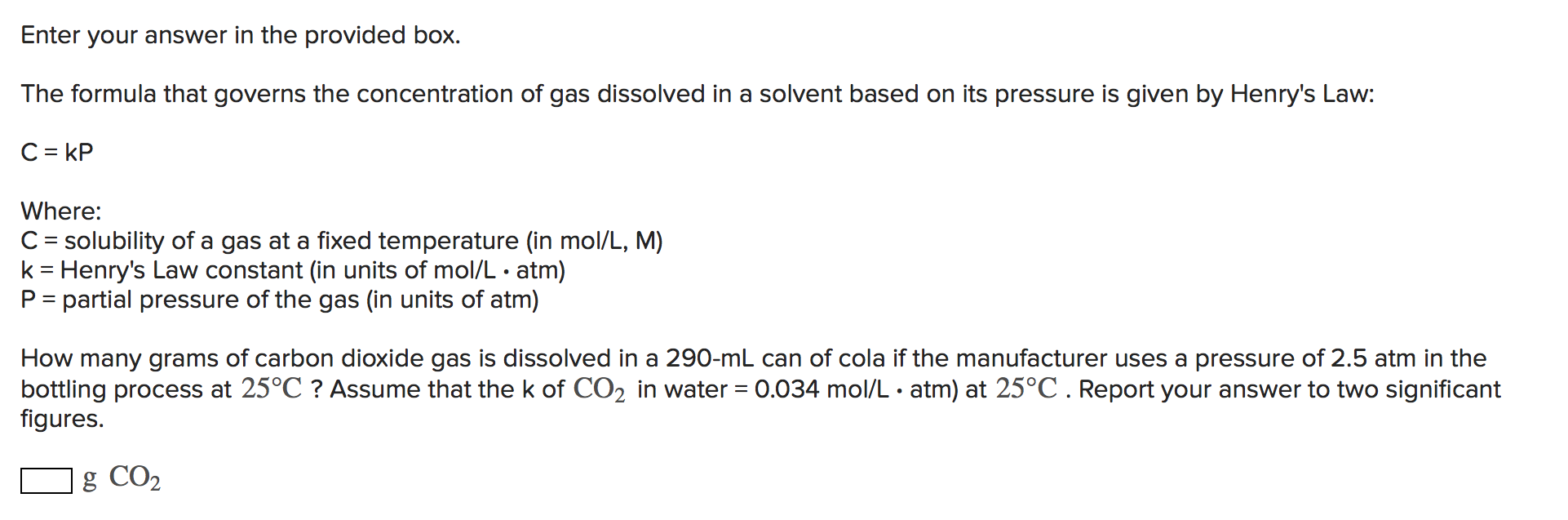Enter your answer in the provided box. The formula that governs the concentration of gas dissolved in a solvent based on its pressure is given by Henry's Law: C= KP Where: C = solubility of a gas at a fixed temperature (in mol/L, M) k= Henry's Law constant (in units of mol/L • atm) P = partial pressure of the gas (in units of atm) How many grams of carbon dioxide gas is dissolved in a 290-ml can of cola...

• ### 12) Write an equation for the dissociation of ethylene, C2H4. Hint: does ethylene dissolve in water? 13) If 0.24 g...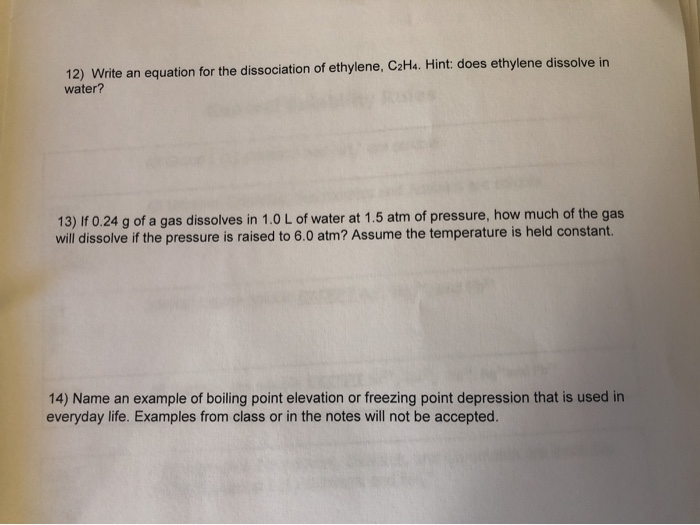12) Write an equation for the dissociation of ethylene, C2H4. Hint: does ethylene dissolve in water? 13) If 0.24 g of a gas dissolves in 1.0 L of water at 1.5 atm of pressure, how much of the gas will dissolve if the pressure is raised to 6.0 atm? Assume the temperature is held constant. 14) Name an example of boiling point elevation or freezing point depression that is used in everyday life. Examples from class or in the notes...

• ### The presence of the radioactive gas radon (Rn) in well water obtained from aquifers that lie in...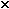(a) Assuming that the solubility of radon in water with 1.00 atm pressure of the gas over the water at 30°C is 7.27 10-3 M, what is the Henry's law constant for radon in water at this temperature?1 mol/L·atm(b) A sample consisting of various gases contains 2.7 10-6mole fraction of radon. This gas at a total pressure of 34 atm is shaken with water at 30°C. Calculate the molar concentration of radon inthe water.2 M

Free Homework App